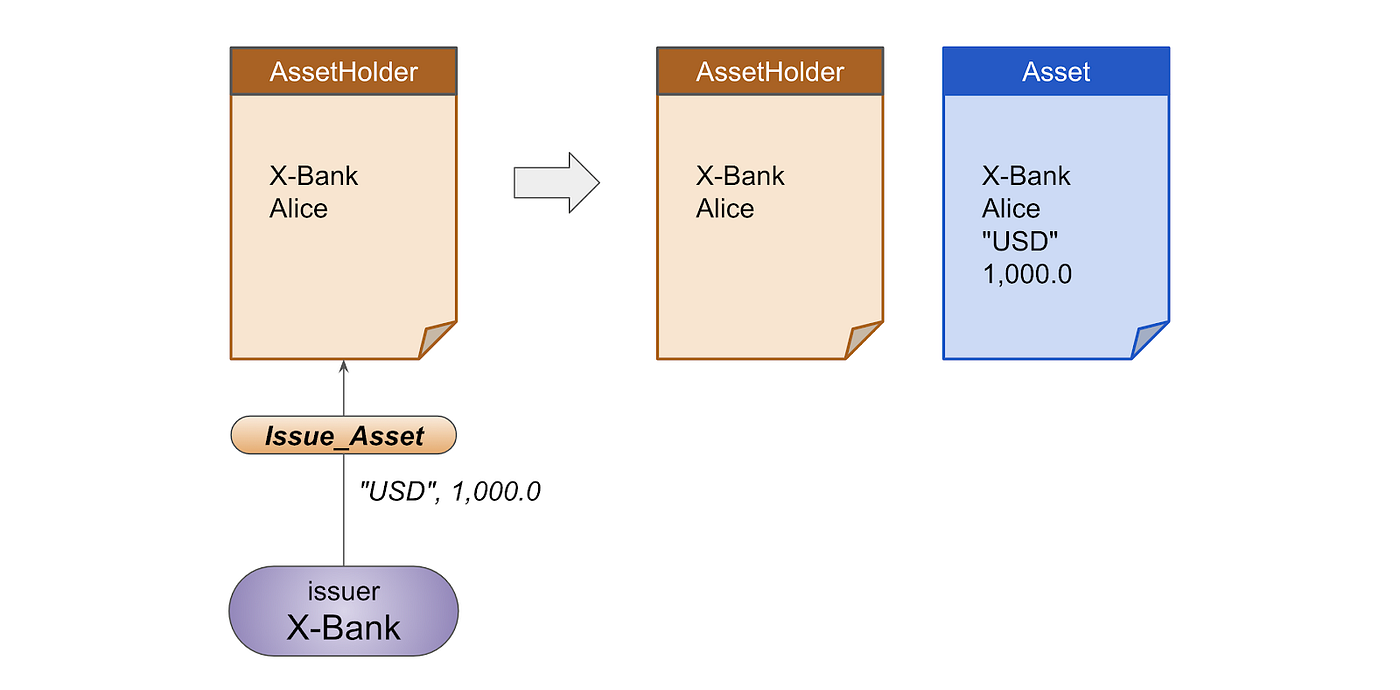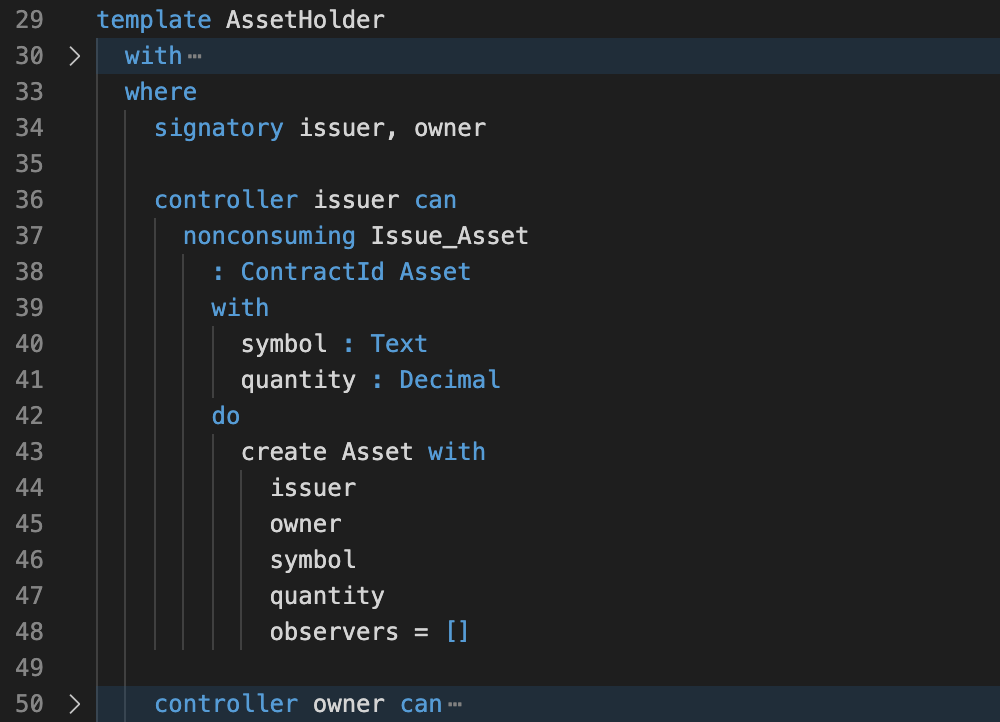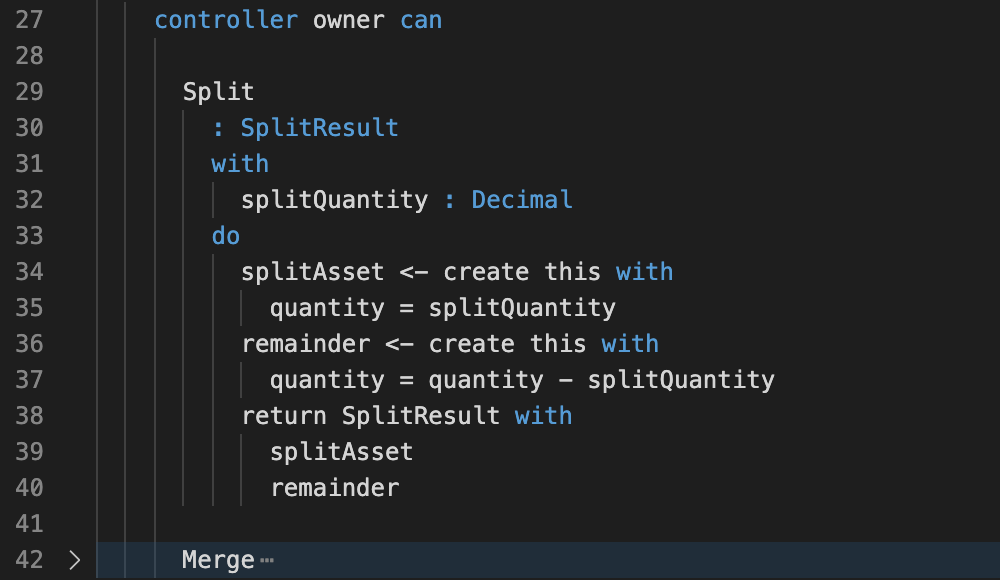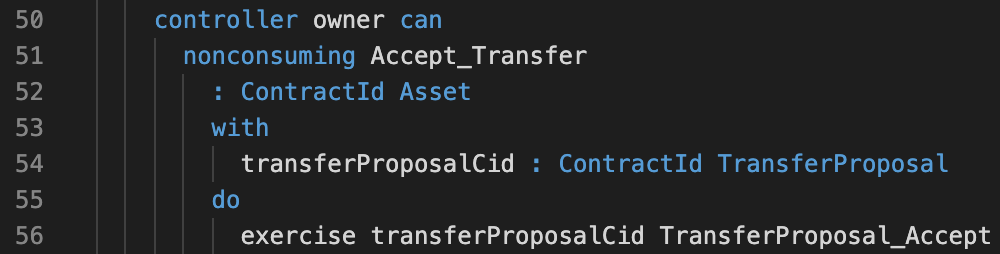# Overview

DAML is the smart contract language modeling digital assets, which can then be deployed in a variety of environments, from databases to distributed ledger platforms such as Hyperledger Fabric and Corda. Their documentation comes with a 10-module tutorial. In my previous articles I talked about the concept of template and contract, and a very common proposal-acceptance model on DAML. They are good for us to get into Tutorial 7, a more comprehensive and well-structured modeling for asset trading. This article is a deep-dive into the Tutorial 7.

In this tutorial we see a more comprehensive and well-structured setup for an asset type.

• The asset requires authority from both issuer and owner (as signatory).
• A role contract (asset holder) exists for each asset owner, facilitating some handling some operations on the assets.
• The asset is fungible.
• The asset is transferable.
• The asset is tradeable, where trading here is an atomic transaction of assets between two parties.

Tutorial 7 is not directly built on top of all previous tutorials, although many ideas are taken from them. The code is well written and carefully designed. By exploring the templates and making some testing scenarios we can learn a lot about DAML coding. Owing to the heaviness of content I make it into two parts. In Part 1 we first inspect the first four items, and leave the asset trading in Part 2.

# Setup

Like my previous works, our focus is on the modeling language itself. We are using DAML Studio with our test script for real-life example simulation If you wish to follow the guide, what you need is to install the DAML SDK (v1.9.0+). Follow the installation instruction here. After installation you can get the code for Tutorial 7 here.

Tutorial 7 comes with some test scripts, all under Test/Intro directory. In this article I have prepared another test script following the flow of the article. It is a step-by-step test script. You can find my test script here. Simply place the test script inside Test/Intro directory (as it is written in the file). You can perform the scenario tests in the document.

# Creation of Asset: Asset Contract and Asset Holder Contract

We first begin with the Asset contract template, from which Asset contracts are created in DAML ledger.

## Asset Contract

Here is the template details about Asset contract (choices are not shown yet).

The information kept in a contract is basic: issuer, owner, symbol of the asset and the quantity. We also see observers as a list of parties, which makes this asset visible to some designated parties.

Both issuer and owner are signatory. This means that the Asset contract is created with authorization from both the issuer and owner. In Tutorial 6 we see how to use the propose-accept model to create this type of asset. In this tutorial a different way is used: through the use of role (i.e. Asset Holder).

## Asset Holder Contracts

In Tutorial 7 a role contract is created for each party by an issuer. We can consider the existence of such contracts as a status of a party, who is an authorized holder of assets issued by an issuer. They are implemented as AssetHolder contracts.

Using role contracts is a more structured way as we can define more action items (choices) which only authorized parties can perform but not others. We will see these choices later.

The information kept in the AssetHolder contract template is quite basic. It only holds both issuer and owner parties (choices not shown yet).

Like Asset contracts, AssetHolder has both issuer and owner as signatory. As we will see very soon, Asset contract is created by issuer exercising a non-consuming choice Issue_Asset in AssetHolder. That is very intuitive as once a party has an AssetHolder status, the issuer can issue as many assets as possible, without going through the propose-accept process every time (like what we have in Tutorial 6)

Having said that, since AssetHolder also requires authority of both issuer and owner (as signatory), we still need the propose-accept model to achieve it. It is the purpose of AssetHolderInvite, which will be created by issuer, and choice AssetHolderInvite_Accept is exercised by the owner to complete the creation of AssetHolder.

This is how AssetHolderInvite contract template looks like, a typical propose-accept model.

With this, our first test script (test1) is to create AssetHolder contract through AssetHolderInvite.

And here are the results. We can see that the AssetHolder contract is created, with both X-Bank and Alice as signatory. The AssetHolderInvite contract is archived after Alice accepts the invitation.

## Asset Contract Creation

As mentioned before, Asset contract requires both issuer and owner as signatory. We see how it can be created through the propose-accept model in Tutorial 6. Here we place the creation of Asset contract as a choice under AssetHolder contract. As AssetHolder bears both issuer and owner as signatory, the newly created Asset contract also obtains authorization from both issuer and owner.

In the AssetHolder contract template, issuing assets is done by the issuer with the choice Issue_Asset. As we can see here, it requires two inputs: symbol and quantity. The result of this choice is an Asset ContractID. The logic is to create an Asset contract based on the details about issuer and owner from the AssetHolder contract, symbol and quantity as input arguments, and finally an empty list for observers.Issuer creates Asset contract through exercising in AssetHolder contract.

Note that it is a non-consuming choice. This contract AssetHolder is still active after this choice is exercised. This makes sense as the issuer can issue multiple assets to an owner through this AssetHolder contract.Choice Issue_Asset in AssetHolder template (Asset/Role.daml)

Here we simulation the creation of Asset with the test script test2. The issuer can issue more than one asset with the same AssetHolder contract.

Note: We use the result of test1 (line 25), such that we do not need to repeat the AssetHolder process. They are used in the remaining tests in Part 1.

And here is the result. You can see X-Bank has issued two Assets to Alice through AssetHolder contract, and this contract is still there for further asset issuance if needed.

# Fungibility of Asset

## Overview

Asset fungibility means that each unit of an asset is indistinguishable from other units. In real life banknotes are fungible, as this one-dollar banknote is of no difference from another one-dollar banknote, as both bear the same value. Real estate is non-fungible assets, as this house is different from another house.

One nature of fungible assets is that it can be grouped together and divided into parts. To show the fungibility of an asset, Asset contract template comes with two choices: Split and Merge. We first take a look at Split.

## SplitChoice Split in Asset template (Asset.daml)

Choice Split can be exercised by the owner of this Asset. When exercising Split, owner provides a splitQuantity value. The result is two newly created Asset contracts, with one holding quantity equal to the splitQuantity (line 34–35), and the other holding the remainder (line 36–37). The result is a data record (SplitResult) storing two Asset contracts (see line 7–10 in `Asset.daml`).

We are using test script test3 to split the asset of USD 1,000.0 into two, with the split quantity USD 300.0.

Here is the result.

We see that at the beginning we have one Asset contract (#2:1) created with 1,000.0. After Alice exercises Split with splitQuantity 300.0, this Asset (#2:1) is archived, and two new Asset contracts are created with one 300.0 (#3:1) and another 700.0 (#3:2).

# Merge

Now the Merge choice.Choice Merge in Asset template (Asset.daml)

Choice Merge can be exercised by the owner of this Asset. When exercising Merge, owner provides an active Asset contract, otherCid, to be merged to the current one. The result is a newly created Asset contract, by computing the sum of quantity from both (line 59 is the logic). Certain protection is needed before merging is done: the two contracts must be of the same issuer, the same owner and the same symbol. The merge happens only if these three conditions are satisfied. And the given otherCid contract needs to be archived after it is being merged to the new Asset Contract.

We are using test script test4 to merge the two Asset contracts.

Here is the result.

Both to-be-merged Asset contracts get archived, and the resulting new Asset contract has the right quantity.

This is the summary of Split and Merge choice in Asset contract.

# Asset Transfer

If you have walked through Tutorial 6, you probably see how the transfer of assets is implemented in the propose-accept model. Tutorial 7 follows this model and involves AssetHolder contracts.

We first see the proposal stage, and after that we will examine the acceptance stage.

## Proposal Stage

The sender, the asset owner, exercises the choice ProposeTransfer in Asset contract template.

The asset owner simply provides the receiver, newOwner. The logic is to create a new TransferProposal contract, with the asset details and receiver. Note that this is a consuming choice, which means that the Asset contract will get archived after ProposeTransfer is exercised. The asset detail is then kept and passed to TransferProposal contract.

Now it’s time to take a look at the TransferProposal contract template.

This is the standard Cancel / Reject / Accept response pattern for transfer proposal, as we have seen in Tutorial 6. We should allow both asset.owner to cancel the proposal (line 94–99) and receiver (newOwner) to reject the proposal (line 101–105), as it is the only way to recover the asset being archived in the previous step. Now we can take a look at the code for acceptance by asset.issuer and newOwner (line 87–92).

## Acceptance Stage

For acceptance, we see that both asset.issuer and newOwner authority needed to exercise the choice TransferProposal_Accept. As a result, the receiver cannot exercise this choice directly, as the authorization asset.issuer is missing.

For demonstration purpose, we simulate the error with test5_1 script and let Bob exercise TransferProposal_Accept directly (line 112–113).

We immediately see the error in the result.

To gain authority of asset.issuer X-Bank, a choice Accept_Transfer is coded in AssetHolder contract. Here is what the choice looks like.Choice Accept_Transfer in AssetHolder contract (Asset/Role.daml)

This is the receiver’s AssetHolder contract. To accept the proposal, the receiver (owner), exercises Accept_Transfer, with a TransferProposal contract ID, created by the sender in the proposal stage. With this the original TransferProposal_Accept is exercised. Here we copy the choice for reference.

The result is an Asset contract, and the logic is simply a creation of this given asset detail with the owner set to the receiver. With the help of AssetHolder contract, now we have both authority of asset.issuer and newOwner (receiver).

## Test Script

We saw the error above. Here we simulate the correct one in test5, We first create Bob’s AssetHolder contract (line 76–82). Then let Bob accept the proposal by exercising Accept_Transfer choice in his AssetHolder contract (line 93–95).

Now the result shows the Asset is successfully transferred to Bob. That is, an active Asset contract with the same content and owner changed to Bob, while Alice’s Asset contract are archived.

We see by exercising the correct choices in the correct contracts, assets are safely transferred between parties.

This shows the difference: Bob cannot accept the TransferProposal directly. Through AssetHolder Bob obtains authority from X-Bank, and the acceptance of TransferProposal is then successful.

# Summary

We have walked through quite a large portion of the code for Tutorial 7. We saw how asset is created through asset holder contracts and how fungible asset is being split and merged. With the help of asset holder contracts we also observed how assets are transferred.

The missing part is asset trading, an atomic transaction of assets between parties. We will show how this is implemented in Part 2.

--

--